# Calculations for Design Parameters of Transformer

Table of Contents

# Design Parameters of Transformer

For Quick review and summary of Calculations, see the tables at the end of this article. (Table 1) (Table 2) For better understandings, go through the following steps for calculations. Make sure you have knowledge of Basics of Transfomer

## Design Parameters

For designing a transformer, we need:

1. Power rating
2. Voltage levels (primary and secondary)
3. Currents on both sides
4. Primary and secondary coils wire diameter/size
5. Iron Core area
6. Numbers of turns (primary and secondary)

We are going to design a 50 VA step down transformer of 230V to 12V. Necessary calculations along with formulae are given below in details:

As we are going to design small transformer (of small power rating) so we are neglecting core and copper losses as they doesn’t matters in small transformers and seriously considered in designing power transformers (high power rating transforms).

## Calculations:

### 1. Core Calculations:

Calculate area of core (central limb) by using following formula: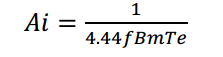Ai= area of core
F= operating frequency
Bm= magnetic flux density
Te= turns per volts
(for derivation of this formula Click Here)

#### Assumptions:

So, we know the frequency of the power system. We need magnetic flux and turns per volts. For designing a small transformer magnetic flux density is averagely taken as 1 to 1.2.
By putting values we will get the area of core.
Current density of copper wire is taken as 2.2 A / mm2 to 2.4 A/ mm2 (approximately).
So, putting values
```F= 50 hz
Bm = 1.2 wb/m2
Te = 4 (turns per volts)```As, we are going to design a practical transformer so we must consider the core available in market. The standard Bobbins available in market practically is 1”x1”, 1.25”x1.5”, 1.5”x1.5” and so on. We took nearest core area available to our calculation. We took bobbin of 2.25 inch2 (1.5”x1.5”) or 0.00145161 meter square.  We have the core area. We can calculate turns per volts using this area by following:
Putting f=50 hz;                Bm = 1.2 wb/(m^2);             Ai= 0.001451 m^2,                 we got: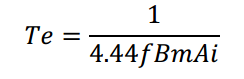So, Turns per volts are 2.6 Turns per volts.

## Primary Winding Calculations

```Primary voltage = Vp = 230 V
Primary current = I1= VA / Vp = 50 / 230 = 0.218

Let transformer we are going to design is 95 % efficient so,
I1 = VA/ (efficiency x Vp) = 0.23A
Primary Current = 0.23 Amp (approx.)```

### Number of Turns:

```Total number of turns = turns per volts x primary side voltage
= 2.6 x 230
N1   = 600 turns (approximately)

```

### Size of Conductor:

As we know that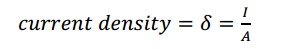As, for copper the current density is taken as 2.3 A per mm square So, for area of copper conductorFrom the standard American wire gauge table, we can choose wire of the same thickness. It can be seen that it comes out that required primary side wire is of 27 gauge which can conduct required current. (for AWG table Click here)

Selection of wire can also be done by calculating primary current and by cross match the standard table of copper wire according to their current handling capabilities.

## Secondary Winding Calculations

```Secondary voltage = Vs = 12 V

Secondary current = Is = VA/ Vs = 50 / 12 = 4.2 Amp (approx.)```

From the standard copper wire, table it can be seen that wire of this thickness is of 15 gauge. So for secondary winding we need 15 gauge wire.

So,

Secondary Wire =15 AWG

### Number of Turns:

`Number of secondary turns = turns per volts x secondary volts`
```                       N2 = 2.6 x 12 = 32 turns (approx.)

```

## Weight Estimation of Windings

For weight calculations, following steps will be followed:

1. Approximate length of copper wire = perimeter of bobbin x number of turns
2. Cross sectional Area of copper conductor
3. Volume = Approximate length x Cross sectional area
4. Mass = density of copper x Volume

Density of copper = 8960 kg/ m3

### Primary side:

```Perimeter of bobbin = (1.75 x 4) = 7 inch  = 0.1778 m

So,

Length of one turn = 0.1778 m
Total length of all turns of primary = L1

L1 = (length of one turn) x(total number of turns of primary)
L1 = 0.1778 x 600
L1 = 106 m (approx.)

As,
area of primary conductor = 0.1 mm2
= (0.1 x 10 ^ -6) m2
Volume of copper wire = area x length```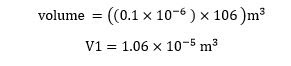And                                        density of copper = 8960 kg/ m3

So,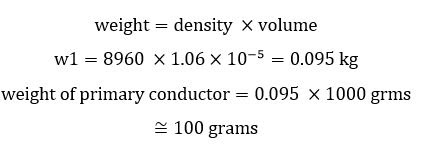So we need approx. 100 grams of 27 gauge wire.

### Secondary winding weight:

```Perimeter of bobbin = (1.75 x 4) = 7 inch = 0.1778 m

So,

Length of one turn = 0.1778 m

Total length of all turns of Secondary

= L2 = length of one turn x total number of turns of secondary

L2 = 0.1778 x 32

L2 = 6 m (approx.)

As,
area of primary conductor = 1.83 mm2 = (1.83 x 10 ^ -6) m2

Volume of copper wire = area x length

```So, we need approx. 100 grams of 15 gauge wire.

So, from above calculations we can summarize in following table:Table 1: Calculation for primary side
---------------------------------------------------------------------------------------------------------------------Table 2: Design Summary for Secondary of transformer design

At this point you have done the Calculations and you have the characteristics of transformer components. Now, for making in hard form, see few easiest steps of hardware implementation of calculations:

Here is another article for transformer core optimization using Genetic Algorithm - a heuristic method of optimization.

For any Queries and further Assistance, feel free to comment below and like our facebook page for new updates.

#### Asad Ullah

I am MSc Scholar in Electrical Engineering. Working with Modeling and Simulation software related to my field are my activities in leisure.

## 109 thoughts to “Calculations for Design Parameters of Transformer”

1. Anonymous says:

Sir.the value of current density is not internationally.that u put.

2. noxuz says:

Hi, you are not taking into account the total mass of the core needed due to it's saturation of magnetic flux Bm

3. I was looking for formulas to calculate transformar for example i have transformar but i want to rewind it with different input and output ac. i couldn't get formulas for it please

4. BASANT KEWAT says:

nicely engineered Design effort

Basant

5. M Adnan says:

what is the formula to calculatre te area of auto transformer?

6. G. says:

You give formula parameters to use that do NOT make any sense then you solve an equation BUT DO NOT Explain how you derived at simplified variable sub totals and then give a resultant. For example: F= 50 hz
Bm = 1.2 wb/m2
Te = 4 (turns per volts)

Ai= 9.384 × 10-4m 2 ~1.45 inch sq. Really? Are you assuming your viewing audience has taken advanced algebra or is space as a premium you can't explain how you did this or are you just too damn lazy to explain how you got there?

AS the saying goes ----it's like listening to one engineer talk another.

1. Mircea says:

There is a formula in "1.Core Calculations." He gives you values for all variables except one. Then he computes the unknown one. I learned to do that when I was 3rd grade in school. Where do you come from?

7. Anonymous says:

hello sir can you make also a calculation for toroidal transformer?

1. Asad Ullah says:

Unfortunately, toroidal transformer calculations are not available currently.

1. g.satheeshkumar says:

sir, you are put the value of Te=4, please explain this briefly. and next stage u derive this as 2.6 turns. how u adapt the Ai value with this formula Te=1/4.44fABm.

1. Asad Ullah says:

Te=4 is not standard but it is kind of standard for small transformer design. Ai is taken according to the standard bobin sizes available in market. so, by using that size, we can calculate the turns per volts.

2. g.satheeshkumar says:

or Te=4 is it standard for all type of step down transformer

8. Anonymous says:

Hello sir, can i apply this on SWG magnetic wire? We dont use here in philippines AWG table. There is a big difference on AWG ang SWG

Thank you

1. Asad Ullah says:

You can calculate the current in each winding and according to maximum current, you can choose wire from wire gauge table of your ease.

Hope you got your answer.

1. Billy Bob says:

If i sent you a nameplate from a transformer do you think you could estimate the copper content of the transformer with much accuracy?

2. Anonymous says:

No difference inche to mm conversion.

9. Den says:

Anyone knows why the efficiency effect is only applied on primary current ?? How about the secondary current?
And also what should I do if I require 50% margin for core area? Why is also the perimeter value is using 1.75 instead of 1.5??

1. Asad Ullah says:

primary side current is responsible for induction in secondary side as well as losses in core for magnetization and de-magnetization. That's why primary side current is considered for efficiency.

Yes, the perimeter should be 1.5 instead of 1.75

2. Asad Ullah says:

1.75 is not wrong (to some level). As we are not taking other parameters into account for winding. So, Doesn't matter here (to this level of design)

10. Olajide Taiwo says:

Thank you for your design of this single phase transformer, all your explanations are very clear and straight to the point.
It will be very nice if you could please let us have also a design for a three phase transformer.
Thanks a lot.

Jide T.

11. Ebenezer Smart says:

thanks a lot sir,
sir, for someone to design a transformer of 10,000 watts, what size of wire will be needed, cause according to your formula the value am getting is excess and I couldn't see the value i got in the wire Gage data sheet

1. Asad Ullah says:

As mentioned in article, this method is good and suitable for designing small transformers. For high power transformer, other factors affects too.
Anyways, just as a tip: for current handling you can use copper strips for winding and use multiple parallel paths for same winding. (i.e. multiple parallel conductor bars to handle. If a bar handles 10Amps max, you can use two parallel bars for 20Amps.) Again, it is just a tip. use on your own risk.
P.S: But the use of strips are valid.

12. Mircea Bujor says:

Hi, Is it ok to use 4A/mm2 current density in a toroidal transformer? I understand your design is for square transformer.

1. Asad Ullah says:

These calculations cum assumptions are for designing of soft silicon steel based core transformers. As far i know, torodial transformers have core of other iron, cobalt, manganese alloy. So, i can't comment about current density for that currently.
Anyhow, That cores requires less energy to energize with higher current densities to handle... exact figure, i can't comment at moment.

13. Abdul Samad says:

Most helpful article.I appreciate your work.Great sir

14. 2x5=10" a\ 60000 fd \ 50hz\ 4.44 gus equatane is t=100000000/4.44*hz*fd*a .... so your t=0.75v
so if you wont 90v taping so 90*0.75=67.5t if you wont 230 v so 230*0.75=172.5t

15. Tebogo says:

Sir help me understand how to do calculations for transformer power rating.How do you decide on the size of your transformer in kVA or MVA?

1. Asad Ullah says:

Power rating of transformer depends on the use of transformer. VA, kVA, MVA depends on your application of transformer.

16. Tebogo says:

Sir what is the shape of this bobbin and which side are you referring to since we used a 1.5inchx1.5inch bobbin?

17. Faizan Ahmad says:

its very help full article.

18. Anonymous says:

GREAT sir!

19. Virendra Singh says:

Above Calculation based on the Value Te=4 Turn Per Volt. What is benefit/drawback if we choose a big/small size of Bobbin

1. Asad Ullah says:

bobbin size is, somewhat mechanical parameter which decides size of your transformer. Greater size bobbin will only increase cost and weight of transformer material. Smaller bobbin will not be able to hold enough steel core for required power transfer (from primary to secondary).

20. Munawar says:

is it possible to put a 500kva transformer winding in another 500kva transformer core ????

1. Asad Ullah says:

if both are designed to serve the same purpose, there is nothing wrong replacing one's winding with other's.

21. VICTOR AGBEBI says:

I need to construct a 1.5kVa transformer with 12-0-12 primary and 220V secondary. What is the wire guage needed and number of turns.kindly help

1. Asad Ullah says:

Just follow the formula given above. Nothing will change except the power ratings. Two windings of same ratings with be there on secondary side.

22. sir, can the size of the copper wire affect the number of turns? if it can, how so? because, as how I understand the solution above, it seems it does not affect. sorry, I'm a newbie. and moreover, we would like to design a 15VA, 220/30 V single phase transformer. we already have 1.25"x1.25" bobbin and a number 25 gauge wire. how can we determine the number of turns?

1. Asad Ullah says:

size of copper wire is effected by the current. Number of turns on other hand, related to voltage (actually to the generation of magnetic flux and ultimately to voltage generation). So, as in article, number of turns are calculated according to voltage requirement and copper wire gauge is calculated by current requirements. Hope you got your answer.

23. Santosh says:

Sir mai 5kva 8no. Core me(input=90v - 300v) automatic stabilizer banana chahata hu to sir mai kaise calculate kr k turn nikalu.

1. Asad Ullah says:

Calculate turn and wire gauge for each voltage level at secondary. Obviously, you want some steps between these voltage levels so there will be as much number of windings as the number of steps of voltages of secondary. Then by the use of relays, voltage sensor and micro-controller programming, you can make this automatic.

24. Santosh says:

Sir mere paas 7no. core(2" * 5") hai to sir mai (input= 90v-300v) tk ka automatic voltage stabilizer ka coil banana chahata hu.
to sir mai kaise calculate kr k turn nikalu.
Plzz bataeye.

25. Anonymous says:

where is the EI iron core detail

26. Guptan Veemboor says:

Thanks for this simple explanation. I am an eighty year old man retired and going through these things just to while away the time. I have some engineering background. But have forgotten everything- practically. Thanks once again.
Regards, Guptan

27. Ralph says:

Does this applicable for multi-winding transformers with different voltage and current rating in every windings.

1. Asad Ullah says:

Yes, if you calculate each winding differently.

28. nadeem butt says:

sir , what is pulse transformer & how to make it .for fence charger input 350-400 volt & output 5000-9000 volt in pulse with 8 mill ampere

29. ndayisaba theoneste says:

i kneed your helper i want to design a step up transformer of 150VA primary voltage is75v and secondary voltage is 220V

1. Asad Ullah says:

Calculations will be same as said in article. just change the voltage levels and you'll get currents and find number of turns and wire gauges accordingly.

30. Anonymous says:

I want to learn about electrical experience more and more.

31. Nooman Khan says:

how to find the bobbin size from power (VA) rating of transformer ?

1. Asad Ullah says:

Actually, VA rating does not have direct affect on bobbin size. It depends on maximum flux density, number of turns and material for core. In this article, we are using laminated stripped iron core and taking standards for maximum flux density and number of volts per turns.
I hope you get your answer.

32. Abhilash Routray says:

F= 50 hz
Bm = 1.2 wb/m2
Te = 4 (turns per volts)
How did u get te =4 turns per volt?

33. inayat says:

Sir kindly answer my question
I made a transformer which have primery winding is 220 turn(18 swg wire 1kg) and secondary turn is 22 turn(10 swg 650gram) now the input voltage is 210 volt and output is 18 volt
bobin size is ( 6inch×3inch) how much current given in output ?????
Plz answer my question

1. Asad Ullah says:

As per calculations, you have current ratio of 1:10 (primary to secondary). Now, as you have 18 SWG so it can have max of 3Amp. So, it can have 3amp to primary and almost 25~30Amp at secondary (at max).

34. I AM CONFUSED BY THE VERY FIRST FORMULA BECAUSE YOU SAY THAT Bm IS MAGNETIC FLUX_Phi_(Wb) AND YET YOU SUBSTITUTE THE MAGNETIC FLUX DENSITY(Wb/m^2) HOW SO???

1. Asad Ullah says:

It is actually magnetic flux density, no matter the nomenclature in the article it is still magnetic flux density.

35. Adnan khan says:

Sir ma transformer banana chatha ho mera pas iron core pare ha his ma 3.8cm5cm ha moja wire no bata dena tarn me tadad be secondary or prembry Dino ma ma an pard ho koch moskil aya gee please ap mer madad kro thanks

1. Asad Ullah says:

You want transformer of which ratings ? Wire size depends on power ratings of transformer.

36. Asad, This page is absolutely fantastic. Thank you very much. In the above, "1.75 is the length of one side of the bobbin." In the example on this page, I understood that a standard size of 1.5 inches by 1.5 inches was used for the example calculations. Could you please help me understand where the 1.75 inches is derived from?

37. shivam patel says:

Sir,I made a transformer 24V 3A,72VA operated in 220V which heated more without load..and output is accurate, bobbin area is 10.26sqcm , primary turn 937 , turn per volt 4.26, secondary turn 106 ,primary wire gauge 28, secondary wire gauge 19 can anyone please explain why so heated?????thanks

1. Asad Ullah says:

Heating could be due to low quality core material or loose binding of core (air between core stampings) as a lot of power is being lost in it. Use laminated core stamping for core and hard bind them to avoid losses.

38. Bobby says:

pls for large transformer power like 6kva, how will i get the value of flux density (Bm)

39. Amabo says:

By using the same perimeter value for both primary and secondary, you implicitly assume that windings are side by side (split bobbin) rather than one over the other. Standard practice is to use not perimeter but mean length of term (MLT), thus the stated wire lengths are too long. The winding area is not filled 100% because the wire is round and has air included in the winding cross section. Standard practice is to use a fill factor which tends to be around 60% when winding technique and insulation thickness are included. This affects the choice of wire gauge to fit the cross section. From wire length and gauge the next step is to calculate resistances from which the voltage drops can be known, noting that AC resistance will be somewhat greater than DC resistance. Then the design can be iterated to compensate.

1. Asad Ullah says:

Yes you are right. I am aware of 'mean length per turn', 'window effective area', resistance of winding, core geometrical constants and many more factors according to which transformer is designed. Here, we have designed small transformer and with compensation factor, some parameters are neglected and some are included in compensation factor.
Thank you for your considerations. Design calculations according to all these factor included will be available soon.

40. behzad says:

as i figured out, all the formulas work only for ac input. what about a rectangular pulse? should i use Fourier expression off voltage and then find EMF equation?

41. Faisal Javaid says:

most help full artical or information....welldone
Asad bhai aap transfomer ka temprature rise find out kr skty hain to kinly mari kuch help kr dain aur L.T ,H.T eddy loss formula k bary ma b bta dain....Thanks

42. Anonymous says:

How to make welding transformer?

43. adeh augustine says:

Mr Asad thank you for your explanation. Please I have a problem similar to this...am asked to find the parameters of a Transformer core (shell type) such as height(H), diameter(D), width(W), width and length of the window slot(Ws). Haven being given insulation thickness as 2mm, V=220/18v, P=450W, power factor as 0.8, window factor as 0.3, maximum flux density(Bm=0.42T), F=50Hz, k=1.2.
Please how can I go about this.

44. Mohammed Idrees says:

What is current density for aluminium? Is the current density value is fixed?

1. Asad Ullah says:

Yes, every material (conductor) have its constant current density as it is property of material. Aluminum has current density of around 0.5 A/mm^2

45. Mohammed Idrees says:

How to design k rated transformer

46. Stanley Izebhokhae says:

bobbin of 2.25 inch2 (1.5”x1.5”) or 0.00145161 meter square. how dis you get 0,00145161!

1. Asad Ullah says:

2.25 inch square = 0.001455161 meter squares
it's simple area units conversion.

47. syed says:

asslamwalkum sir can i u please explaine me the core calculation of CT

1. Asad Ullah says:

CT is a transformer with very low voltage drop at primary side and it is stepped up for measurement. Core calculation for small CTs is almost same as other transformers but for very small sized CTs, ferrite core is used which need different set of calculations.

48. Kushal Trivedi says:

Respected sir,how we find the laminations size if it is NOT C.R.G.O. IT IS C.R.N.G.O

49. Priyanka Naik says:

Thank you for explaining the design of transform. I have one confusion that Perimeter of bobbin=1.75 * 4 you have taken but 1.75 where it comes from???

1. Asad Ullah says:

1.75 is length of one side of bobbin. To calculate length of one complete revolution of wire around bobbin it is multiplied by 4.

50. Syed Hedayat Hussain Zaidi says:

Dear Mr. Asad-ullah Sahab. Your Article is very informative and help full. thanks for sharing it. Regards, May Allah live you long..

51. Idrees says:

How to choose flux density? Whether it wil vary for 3 phase transformers?

52. Akos says:

When you calculate the core area you assumed Te = 4 (turns per volts) but it was not clear where this number 4 comes from. In other calculations the core area is proportional to the squareroot of VA and approximated as Ai = 1.152*sqrt(Output voltage x output current) = 1.152*sqrt(50VA) = 8.146 cm^2=8.146*10^-4 m^2 and then using this Ai value the turns per volt is calculated Te = 1/(4.44AiBf) = 1/(4.44 * 8.146*10^-4m^2 * 1.2T * 50Hz) = 4.6 turns per volts which is close to 4 that you assumed. So actually the turns per volts come from the area and not in the other way because the are comes from the VA. So we don't have to assume anything because everything comes from somewhere.
Apart from this your work is nice. That was the only thing that was not understandable and i had to look it up. Thanks for your post.

1. Asad Ullah says:

That's good thing you took interest and did research in this. But if you look into literature of transformer design, some parameters are constant for specific core size and specific material. You can find standard tables in many books which give you constant values for calculated core sizes. This 4 turns per volt is from standard table for small transformer design calculation listed in Book by "R.K Agarwal" under the title of " Principles of Electric Machine Design"

53. p tirupathi rao says:

for design purpose how much power factor will take for distrubution transformer

1. Asad Ullah says:

For designing a transformer, power factor is not a thing to consider as it depends upon load on transformer. This is one of the important reasons that transformer ratings are in VA (or kVA or MVA).

54. Thank you so much for explaining the parameters of transformers so precisely. My wife was trying to figure out the area of the core; however, did not understand which formula to use. This information definitely makes it easy for us to just plug in our information -- thank you! We did not know that you could just assume the transformers magnetic flux. Thank you again for the information!

55. dhanasekar S says:

NEED 3 PHASE LAMINATED TRANSFORMER CORE WEIGHT CALCULATION FORMULA IN SIMPLE METHOD..?
CAN U PLS HELP ME....?

1. Asad Ullah says:

These calculations are for single phase transformer design. For 3 phase transformer, calculations and formulas are different. (this article will be available soon) 🙂
You can design three phase transformers by connecting three single phase transformers in star. (I personally designed and used it). During connections you must take into considerations phase sequence of all transformers.

56. محترم اسد اللہ صاحب
آج کل آئرن کور کی بجائے گریفائیٹ کور کا استعمال عام ہورہا۔ انرجی سیور میں جو کوائل ہوتی ہے اس سے میں نے ایک 12 وولٹ ٹرانسفر مر بنانا ہے ہے۔ کیا آپ میری مدد کر سکتے ہیں؟ الماس شہزاد

1. Asad Ullah says:

Yes! some times graphite core transformers are used in high frequency applications. Its not something you can generalize about "going out" of iron core transformers.
For that transformers use same calculations for wire of primary and secondary and calculate total area covered by winding according to which you can find out window are and find graphite core of that size.

1. Asad Ullah! Thank to reply. you can guide me further to make step down transformer using ferrite core or give me any link with will be informative for me?

57. Parag rekhi says:

I want calculations for low watts . Ma transformer is pri 220v /sec 1000v and current is 50 ma .
Second transformer is pri 400v sec 5 v and current is 5 ma ...can you help me

1. Asad Ullah says:

Calculations for the wire would be same as for others. If you don't find wire of your desire rating, use smallest ( say 30 AWG wire) to wound your transformer. Your first transformer is of course falls in category of high voltage so lamination precautions must be followed. Likewise, you can design second transformer.

58. loganathan says:

hi... thank u for this article. upto which power rating core & copper losses r neglected..

1. Asad Ullah says:

Mostly, for Calculation of small transformers core losses are neglected (mostly small transformer are considered up to 1000 VA).
However, plus minus 5 percent of turns of secondary winding is added to overcome losses or get full voltage in full load conditions.

59. Andrew says:

Hi, thank you for your article. On the core calculations section, could you explain where you got the 4 turns per volt from that is needed to plug into the equation to calculate the core area. Also, in the same equation, the constant 4.44 - what does this represent? Thank you

1. Asad Ullah says:

For design of small transformer some assumptions have to made. Turns per volts here are from a standard table of designing transformer. (you can study it in book "Principles of Electric Machine Design by R.K Agarwal" or any transformer design book). This is according to transformer type and rating.
4.44 is constant in equation of turns per volts comes while deriving this equation with help of fundamental equations.(click link below for detail derivation).
http://engineerexperiences.com/turns-per-volts-transformer-design.html
Hope you get your answer. 🙂

2. nervic says:

4.44 comes from the rms formula derivation.

60. TYLER BROOKS says:

Hey just wanted to say thanks you for all of these great articles! They read very very well and you've covered all of my questions.

61. ishtiaq says:

At 60 hz frequency what will be flux density

1. Asad Ullah says:

As per formula given in start of article, flux density and frequency are inversely related. But, normally in designing flux density is taken constant (as flux required is constant and area is constant). So, if frequency changes the compensation is made on voltage side. i.e. transformer will be designed accordingly with voltages. but in small transformers such parameters are neglected mostly. (but if you want to, Bm will be constant and voltages will be changed.) P.S: sometimes tolerance voltage compensate this change.

This site uses Akismet to reduce spam. Learn how your comment data is processed.